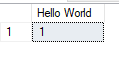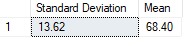## Data Analysis Using R With SQL Server Machine Learning Services

SQL Server Machine Learning Services is an embedded, predictive analytics and data science engine that can execute R and Python scripts within SQL Server as T-SQL script containing R or Python statements, or as R or Python scripts containing T-SQL. The main advantage of it is, the ability to do such analysis where the data resides, eliminating the need to pull data outside the database.

There are two options for SQL Server Machine Learning Services:-

1. SQL Server Machine Learning Services (In-Database) operates within the database engine instance, where the calculation engine is fully integrated with the database engine.

2. SQL Server Machine Learning Server (Standalone) is Machine Learning Server for Windows that runs independently of the database engine. Although you use SQL Server Setup to install the server, functionally, it is equivalent to a non-SQL-Server Microsoft Machine Learning Server for Windows.

In this article, the focus will be on the use of SQL Server Machine Learning Services (In-Database) with R.

### Advantages of SQL Server Machine Learning Services

Machine learning services in SQL Server are designed to provide strong integration with the database engine and the BI stack. Hence, it provides following key benefits to the users.

Security

• As data is kept within the compliance boundary and use of data can be managed and monitored by SQL Server, DBAs have full control over who can access the data.

• DBAs have full control over who can install packages or run scripts on the server. If so desired, they can also delegate permissions on a database level.

Usability

• Developers can use familiar tools such as Management Studio, Visual Studio or even Visual Studio Code to integrate the R or Python code with SQL Server.

• Developers can easily expose the predictive solutions by using stored procedures.

Performance

• Solutions can be optimized using SQL Server technologies, such as columnstore indexes or partitioning, for better performance. Newer features let you batch-train many small models in parallel on partitioned data set, or score millions of rows in using native SQL code optimized for machine learning tasks.

• Developers can do all the analysis where the data resides without pulling data outside the database

### Installation

In the SQL Server setup wizard, On the Feature Selection page, select the following options:

• Select Database Engine Services. (The database engine is required in each instance that uses machine learning).

• Select R Services (In-Database).

• On the Consent to Install Microsoft R Open page, click Accept.

Continue with the setup.

### Enable external script execution

• Execute the following in SSMS
``````sp_configure  'external scripts enabled'
``````

Value of the “run_value” should be 0. (If it is 1, external scripts are already enabled and you can simply skip this step).

• Execute the following script to enable external scripts.
``````sp_configure  'external scripts enabled', 1
RECONFIGURE
``````

Value of the “run_value” should now be 1. External Scripts are enabled and you can start executing R scripts.

Hello World with R and T-SQL

Let’s see how the things are done in SQL Server Machine Learning Services. Execute the following script, which demonstrate the basic building blocks in executing R in SQL server (The same applies to executing Python in SQL Server 2017 as well).

``````EXEC sp_execute_external_script

@language =N'R',

@script=N'OutputDataSet<-InputDataSet',

@input_data_1 =N'SELECT 1 AS hello'

WITH RESULT SETS (([Hello World] int));

GO
``````

Results:-For the first time, it might take some time to generate the results as it takes a little while to load the external script runtime.

Let’s take a look at the query.

• @language parameter defines the language extension to call, in this case, R.

• @script parameter defines the commands passed to the R runtime. Your entire R script must be enclosed in this argument, as Unicode text.

• @input_data_1 is data returned by the query, passed to the R runtime, which returns the data to SQL Server as a data frame.

• WITH RESULT SETS clause defines the schema of the returned data table for SQL Server, adding “Hello World” as the column name, int for the data type.

InputDataSet and OutputDataSet are the default R variables which the input and output datasets are stored retrieved by SQL Server.

### Moving Forward with R in SQL Server

Now, as we know how R scripts are executed in SQL Server, Let’s try to do some simple statistical calculations using R.

Execute the attached StudentMarks.sql which will create a table named StudentMarks with 20 sample records of students’ marks. Using R lets calculate the mean and standard deviation of the marks.

Execute the following script.

``````EXEC sp_execute_external_script

@language =N'R',

@script=N'

InputMarks <- as.matrix(InputDataSet)

sd <- sd(InputMarks)

mean <- mean(InputMarks)

sdFrame <- as.data.frame(sd)

meanFrame <- as.data.frame(mean)

OutputDataSet <- as.data.frame(c(sdFrame, meanFrame))',

@input_data_1 =N'SELECT Mark FROM StudentMarks'  WITH RESULT SETS (([Standard Deviation] numeric(18,2), [Mean] numeric(18,2)))

GO
``````

Results:-Let’s go through the R script line by line to understand what it does.

``````InputMarks <- as.matrix(InputDataSet)
``````

Above code converts the input dataset to a matrix in R and stores in InputMarks variable. SQL Server always sends data as a data.frame. In order to do computations on it, you need to convert it into a vector or a matrix, or any suitable data type.

``````sd <- sd(InputMarks)

mean <- mean(InputMarks)
``````

This is where the actual computation happens. R computes and stores the standard deviation and mean of the dataset, in the variables sd and mean respectively.

``````sdFrame <- as.data.frame(sd)

meanFrame <- as.data.frame(mean)
``````

When your script returns results from R to SQL Server, it must return the data as a data.frame. Any other type of object that you generate in your script — whether that be a list, factor, vector, or binary data — must be converted to a data frame if you want to output it as part of the stored procedure results. The above code does just that.

``````OutputDataSet <- as.data.frame(c(sdFrame, meanFrame))
``````

Finally, the two frames are combined into one and stored in the OutputDataSet which will be returned to T-SQL.

### Moving Forward with R

As you can see, doing standard statistical computations with R is quite easy. Not just statistical computations, R is a very powerful language, which can be used for Analytics, Graphics and Visualizations, Data Mining and Machine learning, etc. You can even install any third party R packages, which provides many advance functionalities, and consume them via SQL Server Machine Learning services.

Here onwards it is all about learning and understanding how R works. There are many R tutorials over the internet. Here are some good places for you to get started.